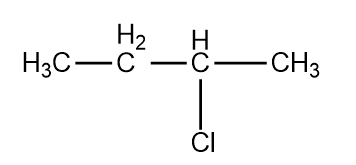#, Cl obtained by chlorination of n-butane, will be                 1. meso form               2. racemic mixture 3. d-form                  4. l-form

Subtopic:  Mechanism of Reactions |
To view explanation, please take trial in the course below.
NEET 2023 - Target Batch - Aryan Raj Singh
To view explanation, please take trial in the course below.
NEET 2023 - Target Batch - Aryan Raj Singh
Launched MCQ Practice Books

Prefer Books for Question Practice? Get NEETprep's Unique MCQ Books with Online Audio/Video/Text Solutions via Telegram Bot

The reactivity order of halides for dehydrohalogenation is

1. R-F>R-Cl>R-Br>R-I

2. R-I>R-Br>R-Cl>R-F

3. R-I>R-Cl>R-Br>R-F

4.  R-F>R-I>R-Br>R-Cl

Subtopic:  Chemical Properties |
To view explanation, please take trial in the course below.
NEET 2023 - Target Batch - Aryan Raj Singh
To view explanation, please take trial in the course below.
NEET 2023 - Target Batch - Aryan Raj Singh
Launched MCQ Practice Books

Prefer Books for Question Practice? Get NEETprep's Unique MCQ Books with Online Audio/Video/Text Solutions via Telegram Bot

In ${\mathrm{S}}_{{\mathrm{N}}^{2}}$ reactions, the correct order of reactivity for the following compounds : ${\mathrm{CH}}_{3}\mathrm{Cl}$${\mathrm{CH}}_{3}{\mathrm{CH}}_{2}\mathrm{Cl}$${\left({\mathrm{CH}}_{3}\right)}_{2}\mathrm{CHCl}$ and ${\left({\mathrm{CH}}_{3}\right)}_{3}\mathrm{CCl}$ is :

1. ${\mathrm{CH}}_{3}\mathrm{Cl}$>${\left({\mathrm{CH}}_{3}\right)}_{2}\mathrm{CHCl}$>${\mathrm{CH}}_{3}{\mathrm{CH}}_{2}\mathrm{Cl}$>${\left({\mathrm{CH}}_{3}\right)}_{3}\mathrm{CCl}$

2. ${\mathrm{CH}}_{3}\mathrm{Cl}$>${\mathrm{CH}}_{3}{\mathrm{CH}}_{2}\mathrm{Cl}$>${\left({\mathrm{CH}}_{3}\right)}_{2}\mathrm{CHCl}$>${\left({\mathrm{CH}}_{3}\right)}_{3}\mathrm{CCl}$

3. ${\mathrm{CH}}_{3}{\mathrm{CH}}_{2}\mathrm{Cl}$>${\mathrm{CH}}_{3}\mathrm{Cl}$>${\left({\mathrm{CH}}_{3}\right)}_{2}\mathrm{CHCl}$>${\left({\mathrm{CH}}_{3}\right)}_{3}\mathrm{CCl}$

4. ${\left({\mathrm{CH}}_{3}\right)}_{2}\mathrm{CHCl}$>${\mathrm{CH}}_{3}{\mathrm{CH}}_{2}\mathrm{Cl}$>${\mathrm{CH}}_{3}\mathrm{Cl}$>${\left({\mathrm{CH}}_{3}\right)}_{3}\mathrm{CCl}$

Subtopic:  Mechanism of Reactions |
To view explanation, please take trial in the course below.
NEET 2023 - Target Batch - Aryan Raj Singh
To view explanation, please take trial in the course below.
NEET 2023 - Target Batch - Aryan Raj Singh
Launched MCQ Practice Books

Prefer Books for Question Practice? Get NEETprep's Unique MCQ Books with Online Audio/Video/Text Solutions via Telegram Bot

Action of RMgX with vinyl chloride gives:

1. alkane

2. alkyne

3. alkene

4. all of these

Subtopic:  Mechanism of Reactions |
To view explanation, please take trial in the course below.
NEET 2023 - Target Batch - Aryan Raj Singh
Launched MCQ Practice Books

Prefer Books for Question Practice? Get NEETprep's Unique MCQ Books with Online Audio/Video/Text Solutions via Telegram Bot

What would be the product formed when 1-bromo-3-chlorocyclobutane reacts with two equivalents of metallic sodium in ether?

1.2.3.4.Subtopic:  Mechanism of Reactions |
To view explanation, please take trial in the course below.
NEET 2023 - Target Batch - Aryan Raj Singh
To view explanation, please take trial in the course below.
NEET 2023 - Target Batch - Aryan Raj Singh
Launched MCQ Practice Books

Prefer Books for Question Practice? Get NEETprep's Unique MCQ Books with Online Audio/Video/Text Solutions via Telegram Bot

$\stackrel{{\mathrm{Cl}}_{2}}{\to }$ Benzotrichloride $\stackrel{\mathrm{Hydrolysis}}{\to }$ Y

X and Y respectievely are:

1. benzene, benzaldehyde

2. toluene, benzaldehyde

3. toluene, benzoic acid

4. benzene, benzoic acid

Subtopic:  Iodoform, Freons & DDT |
To view explanation, please take trial in the course below.
NEET 2023 - Target Batch - Aryan Raj Singh
To view explanation, please take trial in the course below.
NEET 2023 - Target Batch - Aryan Raj Singh
Launched MCQ Practice Books

Prefer Books for Question Practice? Get NEETprep's Unique MCQ Books with Online Audio/Video/Text Solutions via Telegram Bot

Benzene on reaction with a mixture of HNO3 and H2SO4 followed by reaction of Cl2/FeCl3 gives:

1. 3-chloro -1 -nitrobenzene

2. 2-chloro -1 -nitrobenzene

3. 4-chloro -1 -nitrobenzene

4. a mixture of 2-chloro and 4-chloro -1 -nitrobenzene

Subtopic:  Physical Properties |
To view explanation, please take trial in the course below.
NEET 2023 - Target Batch - Aryan Raj Singh
To view explanation, please take trial in the course below.
NEET 2023 - Target Batch - Aryan Raj Singh
Launched MCQ Practice Books

Prefer Books for Question Practice? Get NEETprep's Unique MCQ Books with Online Audio/Video/Text Solutions via Telegram Bot

A yellow precipitate is obtained when aqueous ${\mathrm{AgNO}}_{3}$ is added to a solution of the compound:

1. ${\mathrm{CCl}}_{3}\mathrm{CHO}$

2. ${\mathrm{CHI}}_{3}$

3. ${\mathrm{CHCl}}_{3}$

4. ${\mathrm{C}}_{6}{\mathrm{H}}_{5}{\mathrm{CH}}_{2}\mathrm{Cl}$

Subtopic:  Physical Properties |
To view explanation, please take trial in the course below.
NEET 2023 - Target Batch - Aryan Raj Singh
To view explanation, please take trial in the course below.
NEET 2023 - Target Batch - Aryan Raj Singh
Launched MCQ Practice Books

Prefer Books for Question Practice? Get NEETprep's Unique MCQ Books with Online Audio/Video/Text Solutions via Telegram Bot

In the following sequences of reactions;

${\mathrm{CH}}_{3}{\mathrm{CH}}_{2}{\mathrm{CH}}_{2}\mathrm{I}\stackrel{\mathrm{KOH}\left(\mathrm{alc}.\right)}{\to }$(A)$\stackrel{{\mathrm{Br}}_{2}}{\to }$(B)$\stackrel{{\mathrm{NaNH}}_{2}/{\mathrm{NH}}_{3}}{\to }$(C)

the end product (C) is:

1. alkene

2. alkanol

3. alkyne

4. alkyl amine

Subtopic:  Physical Properties |
To view explanation, please take trial in the course below.
NEET 2023 - Target Batch - Aryan Raj Singh
To view explanation, please take trial in the course below.
NEET 2023 - Target Batch - Aryan Raj Singh
Launched MCQ Practice Books

Prefer Books for Question Practice? Get NEETprep's Unique MCQ Books with Online Audio/Video/Text Solutions via Telegram Bot

Which is gem dihalide?

1. ${\mathrm{CH}}_{3}.{\mathrm{CHBr}}_{2}$
2. ${\mathrm{CH}}_{2}\mathrm{Br}.{\mathrm{CH}}_{2}\mathrm{Br}$
3. ${\mathrm{CH}}_{3}.\mathrm{CHBr}.{\mathrm{CH}}_{2}\mathrm{Br}$
4. None of the above

Subtopic:  Physical Properties |
To view explanation, please take trial in the course below.
NEET 2023 - Target Batch - Aryan Raj Singh
To view explanation, please take trial in the course below.
NEET 2023 - Target Batch - Aryan Raj Singh